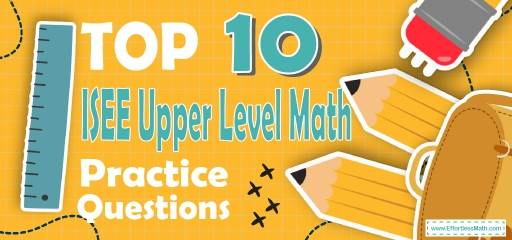# Top 10 ISEE Upper-Level Math Practice QuestionsThe best way to prepare for your ISEE Upper-Level Math test is to work through as many ISEE Upper-Level Math practice questions as possible. Here are the top 10 ISEE Upper-Level Math practice questions to help you review the most important ISEE Upper-Level Math concepts. These ISEE Upper-Level Math practice questions are designed to cover mathematics concepts and topics that are found on the actual test. The questions have been fully updated to reflect the latest 2022 ISEE Upper-Level guidelines. Answers and full explanations are provided at the end of the post.

Start your ISEE Upper-Level Math test prep journey right now with this sample ISEE Upper-Level Math questions.

## ISEE Upper-LevelMath Practice Questions

1- $$\frac{7}{25}$$ is equals to:

A. 0.3

B. 2.8

C. 0.03

D. 0.28

2- The sum of 8 numbers is greater than 240 and less than 320. Which of the following could be the average (arithmetic mean) of the numbers?

A. 30

B. 35

C. 40

D. 45

3- If $$(4.2 + 4.3 + 4.5) x = x$$, then what is the value of $$x$$?

A. 0

B. $$\frac{1}{10}$$

C. 1

D. 10

4- Two dice are thrown simultaneously, what is the probability of getting a sum of 9 or 12?

A. $$\frac{1}{3}$$

B. $$\frac{1}{4}$$

C. $$\frac{1}{6}$$

D. $$\frac{1}{12}$$

5- Simplify :
$$\frac{\frac{1}{2}-\frac{x + 5}{4}}{\frac{x^2}{2}-\frac{5}{2}}$$

A. $$\frac{3 -x }{x^2 – 10}$$

B. $$\frac{3 -x }{2x^2 – 10}$$

C. $$\frac{-3 -x }{2x^2 – 10}$$

D. $$\frac{3 +x }{x^2 – 10}$$

6- Find all values of x for which $$4 x^2 + 14 x + 6 = 0$$

A. $$-\frac{3}{2}, -\frac{1}{2}$$

B. $$-\frac{1}{2}, -3$$

C. $$-2, -\frac{1}{3}$$

D. $$-\frac{2}{3}, \frac{1}{2}$$

7- $$(x + 7) (x + 5)$$ = ?

A. $$x^2 + 12x + 12$$

B. $$2x + 12x + 12$$

C. $$x^2 + 35x + 12$$

D.$$x^2 + 12x + 35$$

8- Which of the following graphs represents the compound inequality $$-2 ≤2x – 4 <8$$?

A.

B.

C.

D.

9- Solve.
$$|9 – (12 ÷ | 2 – 5 |)| = ?$$

A. 9

B. $$-6$$

C. 5

D. $$-5$$

10- Solve: $$2 – 10 ÷ (4^2 ÷ 2) = ?$$

A. 6

B.$$\frac{3}{4}$$

C. $$-1$$

D. $$-2$$

## Best ISEE Upper-Level Math Prep Resource for 2022

1- D
$$\frac{7}{25} = 0.28$$

2- B
$$\frac{240}{8} < x < \frac{320}{8}$$
$$30 < x < 40$$
Then:
Only choice b is correct

3- A
$$(4.2 + 4.3 + 4.5) x = x$$
$$13x = x$$
Then $$x = 0$$

4- D
For sum of 6: (1 & 5) and (5 & 1), (2 & 4) and (4 & 2), (3 & 3), therefore we have 5 options.
For sum of 9: (3 & 6) and (6 & 3), (4 & 5) and (5 & 4), we have 4 options.
To get a sum of 6 or 9 for two dice: $$5 + 4 = 9$$
Since, we have $$6 × 6 = 36$$ total number of options, the probability of getting a sum of 6 and 9 is 9 out of 36 or $$\frac{9}{36}=\frac{1}{4}$$.

5- C
Simplify:
$$\frac{\frac{1}{2}-\frac{x + 5}{4}}{\frac{x^2}{2}-\frac{5}{2}}=\frac{\frac{1}{2}-\frac{x + 5}{4}}{\frac{x^2 – 5}{2}}=\frac{2(\frac{1}{2}-\frac{x + 5}{4})}{x^2 – 5}$$
⇒Simplify:
$$\frac{1}{2} – \frac{x + 5}{4}=\frac{- x – 3}{4}$$
then:
$$\frac{2(\frac{-x – 3}{4})}{x^2 – 5}=\frac{\frac{-x – 3}{2}}{x^2 – 5}=\frac{- x – 3}{2(x^2 – 5)}=\frac{- x – 3}{2x^2 – 10}$$

6- B
$$x_{1,2} =\frac{-b ± \sqrt{b^2-4ac}}{2a}$$
$$ax^2 + bx + c = 0$$
$$4x^2 + 14x + 6 = 0$$
⇒ then: $$a = 4, b = 14$$ and $$c = 6$$
$$x = \frac{-14 + \sqrt {14^2 – 4 .4 .6}}{2 .4} = – \frac{1}{2}$$
$$x = \frac{-14 – \sqrt {14^2 – 4 .4 .6}}{2.4}= – 3$$

7- D
Use FOIL (First, Out, In, Last) method.
$$(x + 7)(x + 5) = x^2 + 5x + 7x + 35 = x^2 + 12x + 3$$

8- D
Solve for $$x$$.
$$-2≤2x-4<8$$⇒ (add 4 all sides) $$-2+4≤2x-4+4<8+4$$
⇒ $$2≤2x<12$$
⇒ (divide all sides by 2) $$1 ≤ x < 6$$
$$x$$ is between 1 and 6. Choice D represent this inequality.

9- A
$$|9 – (12 ÷ | 2 – 5 |)| = |(9-(12÷|-3|))|=|9-(12÷3)|=|9-4|=|5|=5$$

10- C
$$2 – 10 ÷ (4^2 ÷ 2) = 2 – 10 ÷ (16 ÷ 2) = 2 – 10 ÷ (8) = – 8 ÷ 8 = \ – 1$$

Looking for the best resource to help you succeed on the ISEE Upper Level Math test?

## The Best Books to Ace the ISEE Upper-Level Math Test

### What people say about "Top 10 ISEE Upper-Level Math Practice Questions - Effortless Math: We Help Students Learn to LOVE Mathematics"?

No one replied yet.

X
52% OFF

Limited time only!

Save Over 52%

SAVE $40 It was$76.99 now it is \$36.99